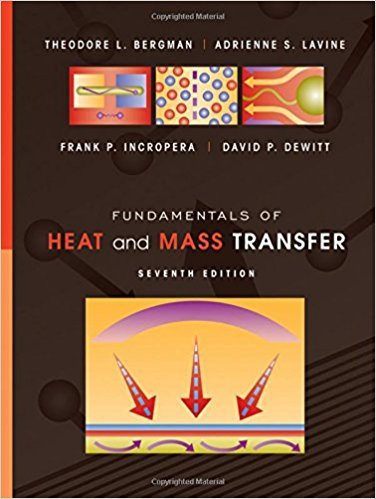×
Get Full Access to Fundamentals Of Heat And Mass Transfer - 7 Edition - Chapter 7 - Problem 7.58
Get Full Access to Fundamentals Of Heat And Mass Transfer - 7 Edition - Chapter 7 - Problem 7.58

×

# A fine wire of diameter Dis positioned across a passagetoISBN: 9780470501979 82

## Solution for problem 7.58 Chapter 7

Fundamentals of Heat and Mass Transfer | 7th Edition

• Textbook Solutions
• 2901 Step-by-step solutions solved by professors and subject experts
• Get 24/7 help from StudySoup virtual teaching assistantsFundamentals of Heat and Mass Transfer | 7th Edition

4 5 1 334 Reviews
14
5
Problem 7.58

A fine wire of diameter Dis positioned across a passageto determine flow velocity from heat transfer character-istics. Current is passed through the wire to heat it, andthe heat is dissipated to the flowing fluid by convection.The resistance of the wire is determined from electricalmeasurements, and the temperature is known from theresistance.(a) For a fluid of arbitrary Prandtl number, develop anexpression for its velocity in terms of the differencebetween the temperature of the wire and the freestream temperature of the fluid.(b) What is the velocity of an airstream at 1 atm and 25C, if a wire of 0.5-mm diameter achieves a tem-perature of 40C while dissipating 35 W/m?

Step-by-Step Solution:
Step 1 of 3

GEOL 207 Environmental Geology More Atmosphere Stuff!! - Albedo  reflection of light on earth. o Clouds  - Air and atmosphere in a system o Circular system (atmosphere)  Much faster than other circular systems such as plate tectonics. - CIRCULAR SYSTEMS (air) o Energy comes from the sun o Buoyancy  The ability or tendency to float in water or air or some other fluid.  Factors  Pressure  Volume  Temperature  If factor (variable) is constant, you can omit it from the equation - Lateral Movement o Sideways movement  Wind travels from high pressure  to low pressure  Air follow pressure gradients - ITCZ  Inter tropical convergence zone o Is the area encircling the earth near the equator where the northeast and southeast trade winds come together o o Coriolis Effect  An effect whereby a mass moving in a rotating system experiences a force (the Coriolis force) acting perpendicular to the direction of motion and to the axis of rotation. On the earth, the effect tends to deflect moving objects to the right in the northern hemisphere and to the left in the southern and is important in the formation of cyclo

Step 2 of 3

Step 3 of 3

##### ISBN: 9780470501979

The full step-by-step solution to problem: 7.58 from chapter: 7 was answered by , our top Engineering and Tech solution expert on 09/04/17, 10:17PM. The answer to “A fine wire of diameter Dis positioned across a passageto determine flow velocity from heat transfer character-istics. Current is passed through the wire to heat it, andthe heat is dissipated to the flowing fluid by convection.The resistance of the wire is determined from electricalmeasurements, and the temperature is known from theresistance.(a) For a fluid of arbitrary Prandtl number, develop anexpression for its velocity in terms of the differencebetween the temperature of the wire and the freestream temperature of the fluid.(b) What is the velocity of an airstream at 1 atm and 25C, if a wire of 0.5-mm diameter achieves a tem-perature of 40C while dissipating 35 W/m?” is broken down into a number of easy to follow steps, and 107 words. This textbook survival guide was created for the textbook: Fundamentals of Heat and Mass Transfer, edition: 7. Fundamentals of Heat and Mass Transfer was written by and is associated to the ISBN: 9780470501979. This full solution covers the following key subjects: Wire, velocity, temperature, heat, fluid. This expansive textbook survival guide covers 14 chapters, and 1569 solutions. Since the solution to 7.58 from 7 chapter was answered, more than 242 students have viewed the full step-by-step answer.

Unlock Textbook Solution